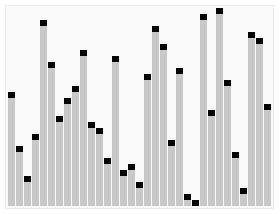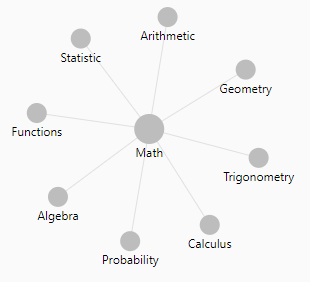# Mathematics - Combination (Binomial coefficient|n choose k)

A combination is a selection of elements from a set where the order of selection does not matter.

Order doesn't matter means that the selections AB and BA are considered a single combination (a single selection).

If the order does matter such as in a digital lock (pin) or the arrival order of a race, the term used is permutation

## Example

• with the set {1,2,3}
• with a selection without_repetition (where the elements are deleted from the set when selected)
• the possible selections are:
• 1 2 3
• 1 3 2
• 2 1 3
• 2 3 1
• 3 1 2
• 3 2 1
• and are all equivalent to the single combination 1 2 3 (because the order in the selections does not matter)

The most known example is a lottery - if the number are selected in the bad order, you still win.

## Formula

### Without repetition

When repeat is not valid (ie AA is not a valid pair)

We say that:

• the elements selected are removed from the set.
• no duplicate element can not be found in a selection.

The best known example of a combination without repetition is lottery numbers (2,14,15,27,30,33)

Combination calculation without repetition is also known as:

• n choose k, because there are $\displaystyle n\choose k$ ways to choose k elements from a set of n elements.
• or Binomial coefficient 1) because it's a coefficient in the binomial theorem

Without repetition, the number of combination possible of length k in a set of possible value of length n is: $$\binom nk = (n \text{ choose } k) = \frac{n(n-1)\dotsb(n-k+1)}{k(k-1)\dotsb1} = \frac{n!}{k!(n-k)!}$$

Note:

• k is also known as:
• the trial number, k = 0, 1, …, n
• the number of elements in each combination
• n is also known as:
• the number total of trial
• the number of element in the whole set

n! is factorial n

Therefore, when the length of the set is equal to the length of the combinations, the number of combinations is 1.

### With repetition

Combination where repetition is allowed is also known as:

• k-selection,
• k-combination with repetition

Example: coins in your pocket (5,5,5,10,10)

There are $\tbinom {n+k-1}k$ ways to choose k elements from a set of n elements if repetitions are allowed.

$$\binom {n+k-1}k = ({n+k-1} \text{ choose } k) = \frac{(k+n-1)!}{k!(n-1)!}$$

## Documentation / Reference

Recommended Pages(Probability|Statistics) - Binomial Distribution

The binomial distribution is the discrete probability distribution of the number of successes in a sequence of n independent yes/no experiments, each of which yields success with probability p. The...Algorithm - Big O Notation

The big-Oh notation is a vocabulary for the design and analysis of algorithms. See: Asymptotic analysis Notation is just . Terminology: running time is O(n...Mathematics - Factorial

The factorial of a non-negative integer x is equal to the product of all integers less than or equal to x and greater than zero. For example: factorial(4) would equal 4 3 2 1, which is 24. In factorial,...Mathematics - Permutation (Ordered Combination)

A permutation is a selection of elements from a set where the order of selection matters. Order matters means that the selections AB and BA are considered as two differents selections. combination ...Python - Combination Codebook

This code can use only numbers. An other processing is needed to apply it to string or symbol. output: With itertools, all characters are allowed. output: Recursive comprehension: Generation...R - Choose (binomial coefficient)

The binomial coefficient n choose 2 is the number n times n minus 1 over 2.Statistics - (Base rate fallacy|Bonferroni's principle)

Every accurate (model|test) can be useless as detection tools if the studied case is sufficiently rare among the general population. The data model will produce too many false positives or false negatives....